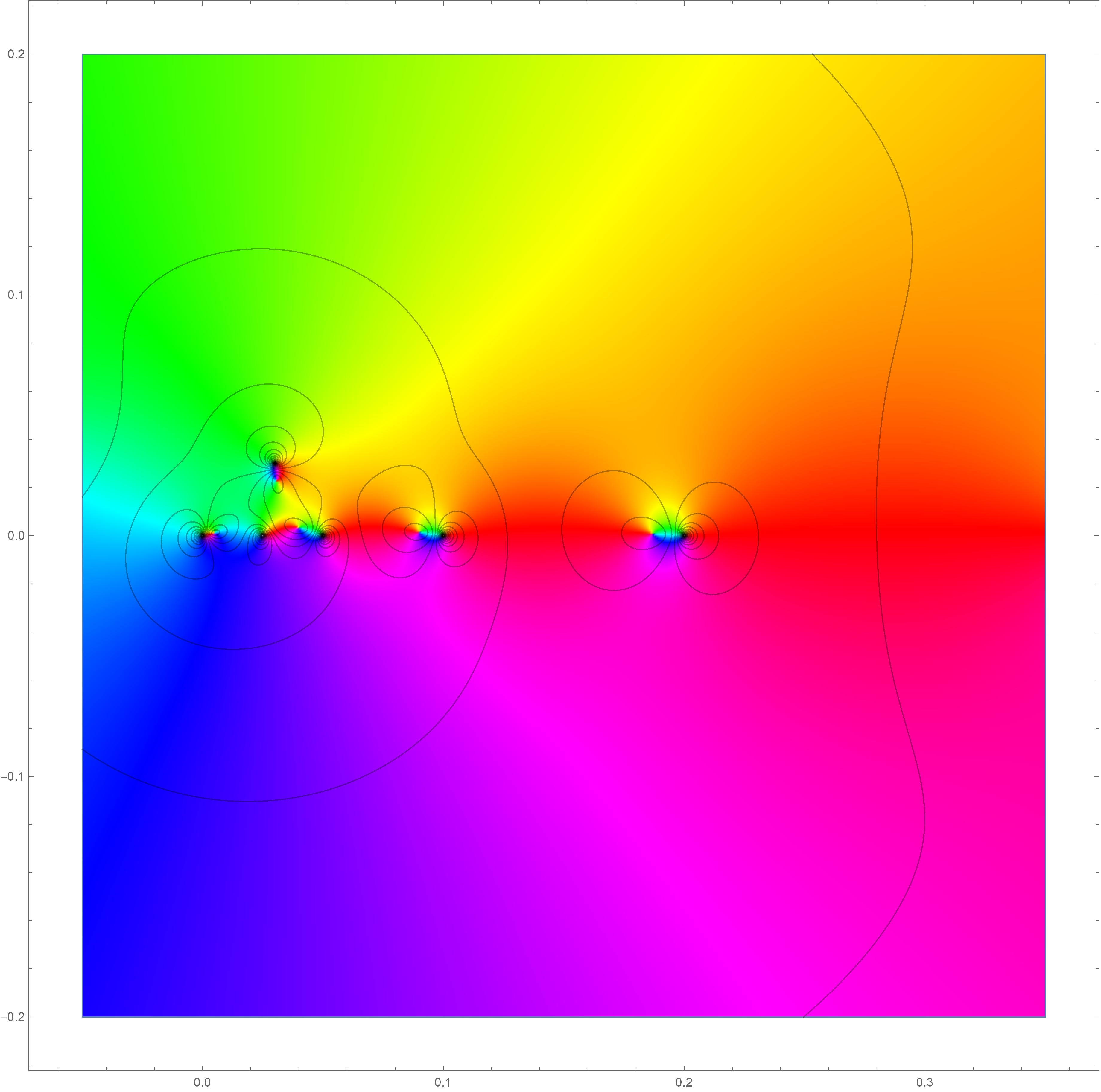# HomeTeachingNotesResearch

My work focuses on the interface between geometric measure theory and otherh areas of analysis. This includes connections to quasiconformal maps and complex dynamics, potential theory and fractal sets, and computational linear algebra. Brief descriptions of each of my publications are below; my preprints are all available on arXiv and detailed summaries of my past and ongoing work are in my research statement.

#### Preprints and Publications (listed chronologically)

1. Improved Hölder continuity of quasiconformal maps, published in Ann. Acad. Sci. Fenn. Math. Quasiconformal maps have certain Hölder continuity properties, among other deep results in their regularity theory. These characteristics are related to some deep questions - for example, it is an open problem to find a (general) way of detecting when a solution to a Beltrami equation is bilipschitz. This is also related to the connectivity of the manifold of chord-arc domains. In this work, I gave a characterization of the Hölder continuity exponent based on a geometric term (via the isoperimetric inequality) and a structural term (depending on the Beltrami coefficient). This approach gives a way to characterize extremizers for Hölder continuity (quantitatively, they must be very close to pure radial stretches). These results generalize to a large class of elliptic equations in the plane.
2. Geometric bounds for Favard length, published in Proc. Amer. Math. Soc. This work involves Favard length and Buffon needle problems in the plane, developing new techniques and estimates that apply to some classes of self-similar sets that arise naturally in fractal geometry. These can be used to give bounds for average projection lengths of sets. This gives an interesting notion of the size of a set (as well as a quantitative measure of rectifiability) that follows classical work of Marstrand and Besicovitch.
3. Stretching and rotation sets of quasiconformal mappings, published in Ann. Acad. Sci. Fenn. Math. Quasiconformal maps have many useful geometric distortion properties; in this work I studied the size (in the sense of Hausdorff measure) of the sets where a given quasiconformal map can have prescribed stretching and rotation; these give quantitative measurements of the continuity of the modulus and argument, respectively. The analysis relies on a highly non-self-similar Cantor set construction (following ideas of my advisor Ignacio Uriarte-Tuero and the work of Lauri Hitruhin) and shows that the distortion sets can be extremely large (from many different perspectives - e.g. gauged Hausdorff measure and Riesz capacity).
4. Commutators of Hilbert transforms along monomial curves, published in Studia Mathematica; joint with Zihua Guo, Ji Li, and Brett Wick. Lp bounds for commutators are frequently used to characterize BMO spaces, going back to classical work of Coifman, Rochberg, and Weiss. For the Hilbert and Riesz transforms (among many other singular integral operators), commutators against a symbol b are bounded precisely when the symbol has bounded mean oscillation. In this work, we study what happens in the case that the operator is the Hilbert transform along a curve. This involves substantial new difficulties, because this operator is singular in a much different way than a typical Calderón-Zygmund operator. We give a partial characterization in terms of a curve-adapted BMO space related to BMO adapted to the parabolic grid.
5. Transversal families of nonlinear projections and generalizations of Favard length, to appear in Analysis & PDE; joint with Krystal Taylor. This work studies nonlinear variants of Favard length, bringing together a consistent set of energy techniques for working with nonlinear projections such as radial projections and the projections associated to Favard curve and Favard surface lengths. We study exactly what geometric properties lead to transversality, and how that can be used to apply energy techniques in many different contexts.
6. Existence of quasiconformal maps with maximal stretching on any given countable set, to appear in Computational Methods and Function Theory; joint with James T. Gill. It's easy to find quasiconformal maps (in any dimension) which have the worst-case regularity at a single point, and this can be extended to any finite set. It turns out that we can extend this to any countably infinite set as well by summing quasiconformal maps with bad stretching (i.e. regularity) behavior at particular points; this technique is rather surprising, because it's very rare that a sum of quasiconformal maps is quasiconformal -- a sum of homeomorphisms isn't even usually injective! The analysis relies on finding the right notion of positivity to work with so that we can unravel the nonlinear quantities which measure volume distortion.Visualization of complex mapping with complicated stretching and rotation at six points in the plane.